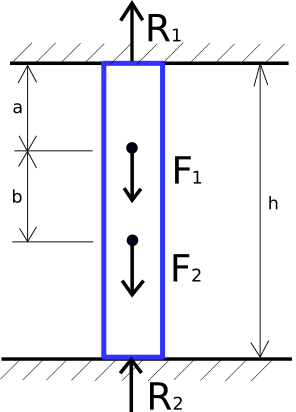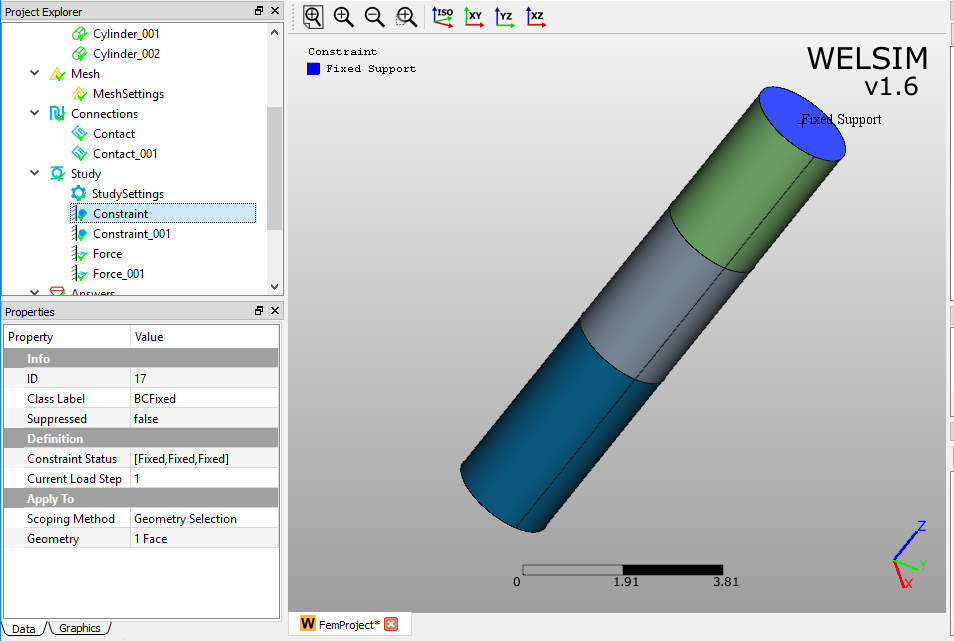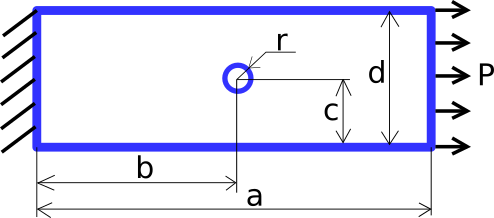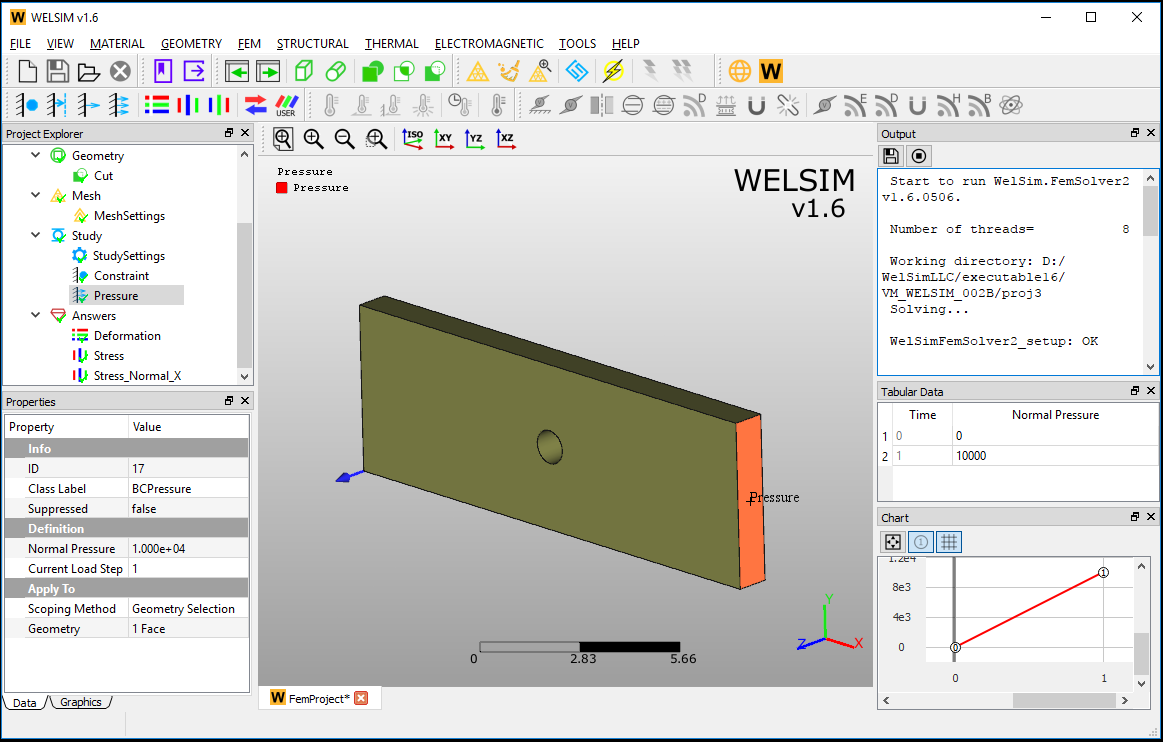# Structural¶

## Statically inteterminate reaction force analysis VM001¶

An assembly of three cylinder bars is supported at both end surfaces. Forces $$F_{1}$$ and $$F_{2}$$ is applied on the middle of the assembly as shown in Figure [fig:ch5_vm_001_schematic].The input data about material, geometry, and loads are given in Table [tab:ch5_vm_001_parameters].

Material Properties Geometric Properties Boundary Conditions
Young's Modulus E=2e11 h=10 $$F_{1}$$=2000
Mass Density $$\rho$$=7850 a=3 $$F_{2}$$=1000
Poission's Ratio v=0.3 b=3

The geometries and imposed boundary conditions are shown in Figure [fig:ch5_vm_001_bc].The result comparison is given in Table [tab:ch5_vm_001_result].

Results Theory WELSIM Error (%)
Z Reaction Force at Top Fixed Support 1800 1810 0.556
Z Reaction Force at Bottom Fixed Support 1200 1202 0.167

This test case project file is located at [vm/VM_WELSIM_001.wsdb].

## Rectangular plate with circular hole subjected to tensile pressure VM002¶

A rectangular plate with a circular hole is fixed along one of the end faces. A tensile pressure load is imposed on another end face as shown in Figure [fig:ch5_vm_002_schematic].The input data about material, geometry, and loads are given in Table [tab:ch5_vm_002_parameters].

Material Properties Geometric Properties Boundary Conditions
Young's Modulus E=2e11 a=15 Pressure P=1e4
Poission's Ratio v=0.3 b=7.5
c=2.5
d=5
thickness=1

The geometries and imposed boundary conditions are shown in Figure [fig:ch5_vm_002_bc].The result comparison is given in Table [tab:ch5_vm_002_result].

Results Theory WELSIM Error (%)
Maximum Normal X Stress 3.125e4 3.156e4 0.992

This test case project file is located at %Installation Directory%/vm/VM_WELSIM_002.wsdb.